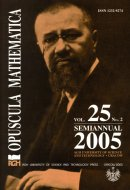Opuscula Math. 25, no. 2 (2005), 319-323

Opuscula Mathematica

# A note on self-complementary 4-uniform hypergraphs

Artur Szymański

Abstract. We prove that a permutation $$\theta$$ is complementing permutation for a $$4$$-uniform hypergraph if and only if one of the following cases is satisfied: (i) the length of every cycle of $$\theta$$ is a multiple of $$8$$, (ii) $$\theta$$ has $$1$$, $$2$$ or $$3$$ fixed points, and all other cycles have length a multiple of $$8$$, (iii) $$\theta$$ has $$1$$ cycle of length $$2$$, and all other cycles have length a multiple of $$8$$, (iv) $$\theta$$ has $$1$$ fixed point, $$1$$ cycle of length $$2$$, and all other cycles have length a multiple of $$8$$, (v) $$\theta$$ has $$1$$ cycle of length $$3$$, and all other cycles have length a multiple of $$8$$. Moreover, we present algorithms for generating every possible $$3$$ and $$4$$-uniform self-complementary hypergraphs.

Keywords: complementing permutation, self-complementary hypergraph, $$k$$-uniform hypergraph.

Mathematics Subject Classification: 34A30, 34B05, 35B12, 35A15, 35J50, 35J65, 46T15, 34B15.

Full text (pdf)

• Artur Szymański
• AGH University of Science and Technology, Faculty of Applied Mathematics, al. Mickiewicza 30, 30-059 Kraków, Poland